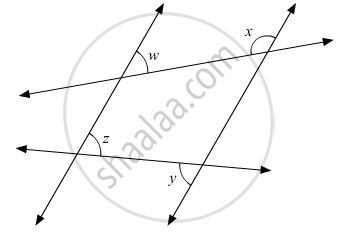# In the Given Figure, If L1 || L2, What Is X + Y In Terms Of W And Z? - Mathematics

MCQ

In the given figure, if l1 || l2, what is x + y in terms of w and z?#### Options

• 180° − w + z

•  180° + w − z

•  180° - w − z

•  180° + wz

#### Solution

The figure is given below:Since, y and z are alternate interior opposite angles. Therefore, these must be equal.

y = z (i)

Also x and are consecutive interior angles.

Theorem states: If a transversal intersects two parallel lines, then each pair of consecutive interior angles are supplementary.

Therefore,

x +w = 180° (ii)

On adding equation (i) and (iii) , we get :

x + y + w = 180°

x + y = 180° + z - w

Concept: Parallel Lines and a Transversal
Is there an error in this question or solution?

#### APPEARS IN

RD Sharma Mathematics for Class 9
Chapter 10 Lines and Angles
Q 15 | Page 54